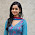## Monday, November 9, 2015

### Time Complexity: Graph & Machine Learning Algorithms

Summary of the time complexity of some common optimization and machine learning algorithms

Overview
Time complexity (or worst case scenario for the duration of execution given a number of elements) is commonly used in computing science. However, you will be hard pressed to find a comparison of machine learning algorithms using their asymptotic execution time.

Summary
The following summary list the time complexity of some of the most common algorithms used in machine learning including, recursive computation for dynamic programming, linear algebra, optimization and discriminative supervised training.

 Algorithm Time complexity Description Recursion with one element reduction N2 N: Number of elements Recursion with halving reduction LogN Recursion with halving reduction and processing N Nearest neighbors search M.logk.N.LogN M: number of featuresk: number of neighborsN: number of observations Matrix multiplication (m,n)x(n,d) m.n.d m,n,d matrices dimension Matrix multiplication (n,n) n3 n matrix dimension Matrix multiplication (n,n)Strassen n2.8 n matrix dimension Partial eigenvalues extraction (n,n) e.N2 e: number of eigenvaluesN: number of observations Complete eigenvalues extraction (n,n) N3 N: number of observations Minimum spanning treePrim linear queue V2 V: number vertices Minimum spanning treePrim binary heap (E + V).LogV E: number of edgesV: number vertices Minimum spanning treePrim Fibonacci heap V.LogV E: number of edgesV: number vertices Shortest paths GraphDijkstra linear sorting V2 V: number of vertices Shortest paths GraphDijkstra binary heap (E + V).logV V: number of vertices Shortest paths GraphDijkstra Fibonacci heap V.log E: number of edgesV: number of vertices Shortest paths kNNGraph - Dijkstra (k + logN).N2 k: number of neighborsN: number of observations Shortest paths kNNGraph - Floyd-Warshall N3 N: number of observations Fast Fourier transform N.LogN N: Number of observations Batched gradient descent N.P.I N: Number of observationsP: number of parametersI: number of iterations Stochastic gradient descent N.P.I N: number of observationsP: Number of variablesI: number of epochs Newton with Hessian computation N3.I N: number of observationsI: number iterations Conjugate gradient N.m.sqrt(k) N: number of observationsm: number of non-zerok condition of the matrix L-BFGS N.M.I N: number of observationsM: estimated number of gradsI: number of iterations K-means (*) C.N.M.I C: Number of clustersM: DimensionN: number observationsI: number of iterations Lasso regularization - LARS(*) N.M.min(N,M) M: DimensionN: number observations Hidden Markov modelForward-backward pass N2.M N: number of statesM: number of observations Multilayer Perceptron (*) n.M.P.N.e n: input variablesM: number hidden neuronsP: number output valuesN: number of observationse: Number of epochs Support vector machine (*)using Newton N3 N: number of observations Support vector machine (*)using Cholesky N2 N: number of observations Support vector machine (*)Sequential minimal optimization N2 N: number of observations Principal Components Analysis (*) M2N + N3 N: Number of observationsM: number of features Expectation-Maximization (*) M2N N: Number of observationsM: number of variables Laplacian Eigenmaps M.logk.N.logN + m.N.k2 + d.N2 N: Number of observationsM: number of variables Genetic algorithms P.logP.I.C C: number of genes/chromosomeP: population sizeI: Number of iterations
(*): Training

References
Introduction to Algorithms T. Cormen, C. Leiserson, R. Rivest - MIT Press 1990
Machine Learning: A probabilistic Perspective K. Murphy - MIT Press, 2012
Big O cheat sheet

1.2.